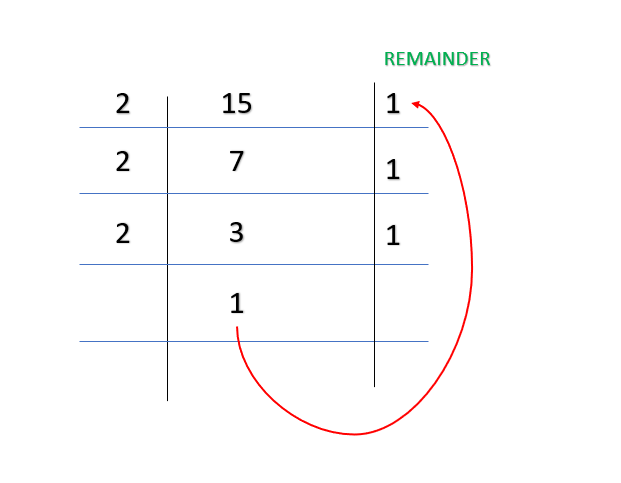# Python program to print the binary value of the numbers from 1 to N

Given a positive number N, the task here is to print the binary value of numbers from 1 to N. For this purpose various approaches can be used.

The binary representation of a number is its equivalent value using 1 and 0 only. Example for k = 15, binary value is 1 1 1 1WORKING FOR METHOD 1

### Method 1: Using the Elementary method with recursion.

Approach

• Divide k by 2.
• Recursive call on the function and print remainder while returning from the recursive call.
• Repeat the above steps till the k is greater than 1.
• Repeat the above steps till we reach N

Program:

## Python3

 `# code to print binary values of first 5 numbers ` ` `  `# recursive function ` `def` `Print_Binary_Values(num): ` `    ``# base condition ` `    ``if``(num > ``1``): ` `        ``Print_Binary_Values(num ``/``/` `2``) ` `    ``print``(num ``%` `2``, end``=``"") ` ` `  ` `  `# driver code ` `if` `__name__ ``=``=` `"__main__"``: ` `    ``N ``=` `5` ` `  `    ``# looping N times ` `    ``for` `i ``in` `range``(``1``, N``+``1``): ` `        ``Print_Binary_Values(i) ` `        ``print``(end``=``" "``) `

Output

1 10 11 100 101

### Method 2: Using Bitwise Operator.

Approach

• Check if k > 1
• Right shift the number by 1 bit and perform a recursive call on the function
• Print the bits of number
• Repeat the steps till we reach N

Program:

## Python3

 `# code to print binary values of first 5 numbers ` ` `  `# recursive function ` ` `  ` `  `def` `Print_Binary_Values(num): ` ` `  `    ``# base condition ` `    ``if``(num > ``1``): ` `        ``Print_Binary_Values(num >> ``1``) ` `    ``print``(num & ``1``, end``=``"") ` ` `  ` `  `# driver code ` `if` `__name__ ``=``=` `"__main__"``: ` `    ``N ``=` `5` ` `  `    ``# looping N times ` `    ``for` `i ``in` `range``(``1``, N``+``1``): ` `        ``Print_Binary_Values(i) ` `        ``print``(end``=``" "``) `

Output

1 10 11 100 101

### Method 3: Using Inbuilt Library of Python

bin() is an inbuilt python function that can convert any decimal number given to it as input to its equivalent binary.

Syntax:

bin (number)

here number is the decimal number that gets converted to binary

Program

## Python3

 `# code to print first 5 binary number using builtIn library ` ` `  ` `  `def` `Print_Binary_Number(num): ` `    ``for` `i ``in` `range``(``1``, num``+``1``): ` ` `  `        ``# using bin to print binary value ` `        ``print``(``int``(``bin``(i).split(``'0b'``)[``1``]), end``=``" "``) ` ` `  ` `  `# driver code ` `if` `__name__ ``=``=` `"__main__"``: ` `    ``num ``=` `5` `    ``Print_Binary_Number(num) `

Output

1 10 11 100 101

Whether you're preparing for your first job interview or aiming to upskill in this ever-evolving tech landscape, GeeksforGeeks Courses are your key to success. We provide top-quality content at affordable prices, all geared towards accelerating your growth in a time-bound manner. Join the millions we've already empowered, and we're here to do the same for you. Don't miss out - check it out now!

Previous
Next